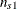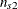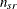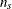# The CATMOD Procedure

### Computational Method

Subsections:

The notation used in PROC CATMOD differs slightly from that used in the literature. The following table provides a summary of the basic dimensions and the notation for a contingency table. See the section Computational Formulas for a complete description.

#### Summary of Basic Dimensions

 s = number of populations or samples (= number of rows in the underlying contingency table) r = number of response categories (= number of columns in the underlying contingency table) q = number of response functions computed for each population d = number of parameters

#### NotationDenotes a column vector of 1s.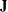Denotes a square matrix of 1s.
Denotes the sum over all the possible values of k.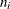Denotes the row sum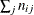.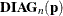Denotes the diagonal matrix formed from the first n elements of the vector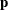.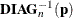Denotes the inverse of.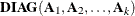Denotes a block diagonal matrix with the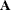matrices on the main diagonal.

Input data can be represented by a contingency table, as shown in Table 32.7.

Table 32.7: Input Data Represented by a Contingency Table

Response

Population

1

2r

Total

1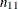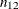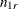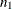2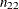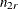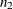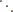s